# Resources tagged with: Exponential and Logarithmic Functions

Filter by: Content type:
Age range:
Challenge level:

### There are 25 results

Broad Topics > Coordinates, Functions and Graphs > Exponential and Logarithmic Functions### Exponential Trend

##### Age 16 to 18 Challenge Level:

Find all the turning points of y=x^{1/x} for x>0 and decide whether each is a maximum or minimum. Give a sketch of the graph.### Function Pyramids

##### Age 16 to 18 Challenge Level:

A function pyramid is a structure where each entry in the pyramid is determined by the two entries below it. Can you figure out how the pyramid is generated?### Integral Equation

##### Age 16 to 18 Challenge Level:

Solve this integral equation.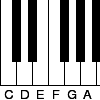### Tuning and Ratio

##### Age 16 to 18 Challenge Level:

Why is the modern piano tuned using an equal tempered scale and what has this got to do with logarithms?### Gosh Cosh

##### Age 16 to 18 Challenge Level:

Explore the hyperbolic functions sinh and cosh using what you know about the exponential function.### Infinite Continued Fractions

##### Age 16 to 18

In this article we are going to look at infinite continued fractions - continued fractions that do not terminate.### How Does Your Function Grow?

##### Age 16 to 18 Challenge Level:

Compares the size of functions f(n) for large values of n.### What Are Complex Numbers?

##### Age 16 to 18

This article introduces complex numbers, brings together into one bigger 'picture' some closely related elementary ideas like vectors and the exponential and trigonometric functions and. . . .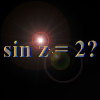### Complex Sine

##### Age 16 to 18 Challenge Level:

Solve the equation sin z = 2 for complex z. You only need the formula you are given for sin z in terms of the exponential function, and to solve a quadratic equation and use the logarithmic function.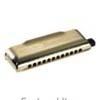### Harmonically

##### Age 16 to 18 Challenge Level:

Is it true that a large integer m can be taken such that: 1 + 1/2 + 1/3 + ... +1/m > 100 ?### Power Match

##### Age 16 to 18 Challenge Level:

Can you locate these values on this interactive logarithmic scale?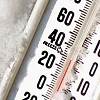### Ph Temperature

##### Age 16 to 18 Challenge Level:

At what temperature is the pH of water exactly 7?### Blood Buffers

##### Age 16 to 18 Challenge Level:

Investigate the mathematics behind blood buffers and derive the form of a titration curve.### Cobalt Decay

##### Age 16 to 18 Challenge Level:

Investigate the effects of the half-lifes of the isotopes of cobalt on the mass of a mystery lump of the element.### Drug Stabiliser

##### Age 16 to 18 Challenge Level:

How does the half-life of a drug affect the build up of medication in the body over time?### Prime Counter

##### Age 16 to 18 Challenge Level:

A short challenge concerning prime numbers.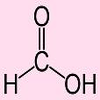### Extreme Dissociation

##### Age 16 to 18 Challenge Level:

In this question we push the pH formula to its theoretical limits.### Log Attack

##### Age 16 to 18 Challenge Level:

Solve these equations.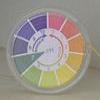### Mixing Ph

##### Age 16 to 18 Challenge Level:

Use the logarithm to work out these pH values### Sierpinski Triangle

##### Age 16 to 18 Challenge Level:

What is the total area of the triangles remaining in the nth stage of constructing a Sierpinski Triangle? Work out the dimension of this fractal.### What Do Functions Do for Tiny X?

##### Age 16 to 18 Challenge Level:

Looking at small values of functions. Motivating the existence of the Taylor expansion.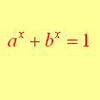### Equation Attack

##### Age 16 to 18 Challenge Level:

The equation a^x + b^x = 1 can be solved algebraically in special cases but in general it can only be solved by numerical methods.### Big, Bigger, Biggest

##### Age 16 to 18 Challenge Level:

Which is the biggest and which the smallest of $2000^{2002}, 2001^{2001} \text{and } 2002^{2000}$?### Hyperbolic Thinking

##### Age 16 to 18 Challenge Level:

Explore the properties of these two fascinating functions using trigonometry as a guide.### Quorum-sensing

##### Age 16 to 18 Short Challenge Level:

This problem explores the biology behind Rudolph's glowing red nose.